Custom SearchTRANSFORMATION FROM POLAR TO CARTESIAN COORDINATES To transform to an equation in Cartesian or rectangular coordinates from an equation in polar coordinates, use the following equations, which can be derived from figure 2-22:Figure 2-22.-Polar to cartesian relationship. EXAMPLE: Change the equation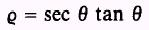to an equation in rectangular coordinates SOLUTION: Applying relations (2.14), (2.15), and (2.16) to the above equation gives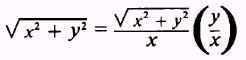Dividing both sides bywe obtain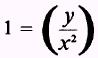or y=x2 which is the equation we set out to find. EXAMPLE: Change the following equation to an equation in rectangular coordinates:SOLUTION: Written without a denominator, the polar equation is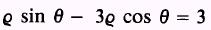Using the transformations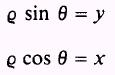we have y-3x=3 as the equation in rectangular coordinates.